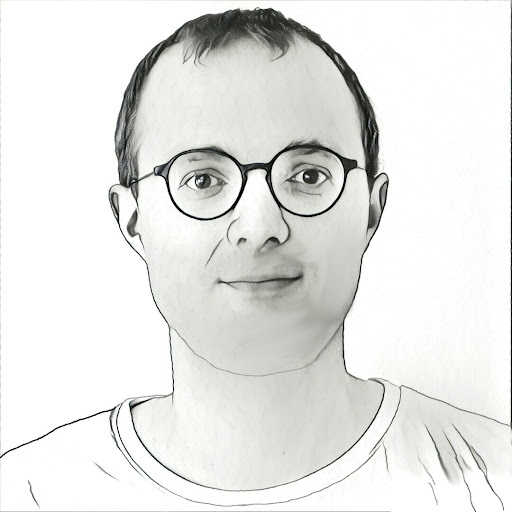Continuous can beat discrete

Nisheeth Vishnoi is a professor at Yale University in the computer science department. The faculty there is impressive and includes many of the top researchers in the world. The CS faculty is pretty good too. As Nisheeth’s PhD advisor, years ago, I am proud that he is at Yale.

Today I wish to discuss a new book by Nisheeth.

The title is Algorithms for Convex Optimization. Let me jump ahead and say that I like the book and especially this insight:

One way to solve discrete problems is to apply continuous methods.

This is not a new insight, but is an important one. Continuous math is older than discrete and often is more powerful. Some examples of this are:${\bullet}$ Analytic number theory is based on the behavior of continuous functions. Some of the deepest theorems on prime numbers use such methods. Think of the Riemann zeta function$\displaystyle \zeta(s) = \sum_{n=1}^\infty \frac{1}{n^s} = \frac{1}{1^s} + \frac{1}{2^s} + \frac{1}{3^s} + \cdots$

as a function of complex numbers${s}$.${\bullet}$ Additive number theory is based on the behavior of continuous functions. Think of generating functions and Fourier methods.

The power of continuous methods is one that I sometimes forget. Nisheeth’s book is a testament to the power of this idea.

## Convexity

Nisheeth’s book uses another fundamental idea from complexity theory. This is: restrict problems in some way. Allowing too large a class usually makes complexity high. For example, trees are easier in general than planar graphs, and sparse graphs are easier than general graphs. Of course “in general” must be controlled, but restricting the problem types does often reduce complexity.

Convexity adds to this tradition since convex generalizes the notion of linear. And convex problems of all kinds are abundant in practice, abundant in theory, and are important.

The MW dictionary says convex means:

: being a continuous function or part of a continuous function with the property that a line joining any two points on its graph lies on or above the graph.

Here is a passage by Roman Dwilewicz on the history of the convexity concept:

It was known to the ancient Greeks that there are only five regular convex polyhedra.

It seems that the first more rigorous definition of convexity was given by Archimedes of Syracuse, (ca 287 – ca 212 B.C.) in his treatise: On the sphere and cylinder.

These definitions and postulates of Archimedes were dormant for about two thousand years!

I say it’s lucky that Archimedes was not up for tenure.

## Nisheeth’s Book

Nisheeth’s book is now available at this site. I have just started to examine it and must say I like the book. Okay, I am not an expert on convex algorithms, nor am I an expert on this type of geometric theory. But I definitely like his viewpoint. Let me explain in a moment.

First I cannot resist adding some statistics about his book created here:

No way I can read the book in nine hours. But I like seeing how many characters and so on the book has. I will have to calculate the same for other books.

## Discrete vs Continuous Methods

Nisheeth in his introduction explains how continuous methods help in many combinatorial problems, like finding flows on graphs. He uses the${s}$${t}$ flow problem as his example. The${s}$${t}$-maximum flow problem arises in real-world scheduling problems, but is also a fundamental combinatorial problem that can be used to find a maximum matching in a bipartite graph, for example.

Combinatorial algorithms for the maximum flow problem. He points out that by building on the Ford-Fulkerson method, various polynomial-time results were proved and other bounds were improved. But he states that the improvements stopped in 1998. Discrete methods seem to be unable to improve complexity for flow problems.

Starting with the paper by Paul Christiano, Jonathan Kelner, Aleksander Mądry, Daniel Spielman, Shang-Hua Teng
the last decade has seen striking progress on the${s}$${t}$ maximum flow problem. One of the keys to this success has been to abandon combinatorial approaches and view the${s}$${t}$ maximum flow problem through the lens of continuous optimization.

Thus, at this point it may seem like we are heading in the wrong direction. We started off with a combinatorial problem that is a special type of a linear programming problem, and here we are with a nonlinear optimization formulation for it. Thus the questions arise: which formulation should we chose? and, why should this convex optimization approach lead us to faster algorithms?

Indeed.

## Open Problems

Take a look at Nisheeth’s site for the answers.

I wish I were better informed about continuous methods in general. They are powerful and pretty. Maybe I could solve an open problem that I have thought about if I knew this material better. Hmmm. Maybe it will help you solve some open problem of your own. Take a look at his book.

[Edited]

1.